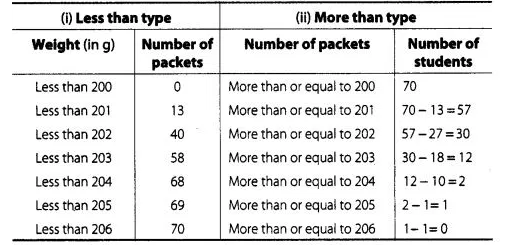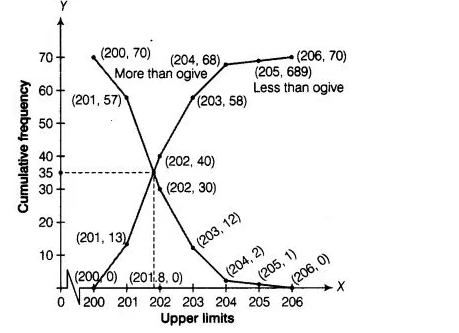# Refer to Q.5 above. Draw the less than type

Question:

Refer to Q.5 above. Draw the less than type and more than type ogives for the data and use them to find the meadian weight.

Solution:

For less than type table we follow the Q.5.

Here, we observe that, the weight of all 70 packets is more than or equal to 200. Since, 13 packets lie in the interval 200-201. So, the weight of 70

-13 = 57 packets is more than or equal to 201. Continuing in this manner we will get remaining more than or equal to 202, 203, 204, 205 and 206.To draw the less than type ogive, we plot the points (200, 0), (201,13), (202, 40), (203, 58), (204, 68), (205,69), (206, 70) on the paper and join

them by free hand.

To draw the more than type ogive plot the points (200, 70), (201, 57), (202, 30), (203, 12), (204, 2), (205,1), (206, 0) on the the graph paper and

join them by free hand.Hence,required median weight = intersection point of x – axis = 201.8 g.